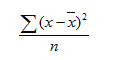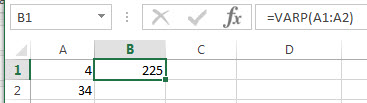# Excel VARP Function

This post will guide you how to use the VARP function with syntax and examples in Microsoft excel.

### Description

The Excel VARP function returns the variance of a population based on an entire population of numbers. This function has been replaced by the VAR.P function in Excel 2010. The variance is used to identify the amount that the values vary from the average value across a set of values. And if you want to calculate the variance for a sample of the population, you can use the VAR function.

The VARP function is a build-in function in Microsoft Excel and it is categorized as a Statistical Function.

The VARP function is available in Excel 2016, Excel 2013, Excel 2010, Excel 2007, Excel 2003, Excel XP, Excel 2000, Excel 2011 for Mac.

### Syntax

The syntax of the VARP function is as below:

`=VARP(number1,[number2],...)`

Where the VAR function arguments are:

• number1-This is a required argument.  The first number or cell reference in a sample of a population.
• number2 -This is an optional argument.  Number arguments 2 to 255 corresponding to a sample of a population. And it can only accept up to 30 number arguments in Excel 2003 or older.

Note:

• The argument must be numbers or arrays or cell references that contain only numbers.
• The VARP function is only used for its arguments are the entire population, and if the argument represents a sample of the population, you need to use the VAR function to calculate the variance.
• If the argument contain the logical values and text representations of numbers and those values will be counted.
• Arguments that are error values or text that cannot be translated into numbers cause errors.
• VARP uses the following formula:### Excel VARP Function Examples

The below examples will show you how to use Excel VARP Function to calculate the variance for data that represents the entire population.

1# to get variance of an entire population of data, using the following formula:

`=VARP(A1:A2)`### Related Functions

• Excel VARA Function
The Excel VARA function returns the variance of a population based on a sample of numbers, text, or logical values. The syntax of the VARA function is as below:= VARA (value1, [value2], ..)…
• Excel VAR Function
The Excel VAR function returns the variance of a population based on a sample of numbers. This function has been replaced by the VAR.S function in Excel 2010. The syntax of the VAR function is as below:=VAR(number1,[number2],…)…
• Excel VARPA Function
The Excel VARPA function returns the variance of a population based on an entire population of numbers, text, or logical values. The syntax of the VARPA function is as below:= VARPA (value1, [value2], ..)…
Related Posts

Excel DVAR Function

This post will guide you how to use Excel DVAR function with syntax and examples in Microsoft excel. Description The Excel DVAR Function will get the variance of a population based on a sample of numbers in a column in ...

Excel DSUM Function

This post will guide you how to use Excel DSUM function with syntax and examples in Microsoft excel. Description The Excel DSUM Function will add the numbers in a column or database that meets a given criteria. And so you ...

Excel DSTDEVP Function

This post will guide you how to use Excel DSTDEVP function with syntax and examples in Microsoft excel. Description The Excel DSTDEVP returns the standard deviation of a population based on the entire population of numbers in a column or ...

Excel DSTDEV Function

This post will guide you how to use Excel DSTDEV function with syntax and examples in Microsoft excel. Description The Excel DSTDEV returns the standard deviation of a population based on a sample of numbers in a column or database ...

Excel DMIN Function

This post will guide you how to use Excel DMIN function with syntax and examples in Microsoft excel. Description The Excel DMIN returns the minimum value from a database or a column of a list that matches the specified conditions. ...

Excel DPRODUCT Function

This post will guide you how to use Excel DPRODUCT function with syntax and examples in Microsoft excel. Description The Excel DPRODUCT returns the product of values from a set of records that match criteria that you specify. The DPRODUCT ...

Excel XIRR Function

This post will guide you how to use Excel XIRR function with syntax and examples in Microsoft excel. Description The Excel XIRR function returns the internal rate of return for a series of cash flows that is not necessarily periodic. ...

Excel VDB Function

This post will guide you how to use Excel VDB function with syntax and examples in Microsoft excel. Description The Excel VDB function calculates the depreciation of an asset for a specified period based on the double declining balance method. ...

Excel SYD Function

This post will guide you how to use Excel SYD function with syntax and examples in Microsoft excel. Description The Excel SYD function calculates the sum-of-years’digits depreciation of an asset for a specified period. And it will return a numeric ...

Excel SLN Function

This post will guide you how to use Excel SLN function with syntax and examples in Microsoft excel. Description The Excel SLN function calculates the depreciation of an asset for one period based on the straight line depreciation. And it ...

Sidebar